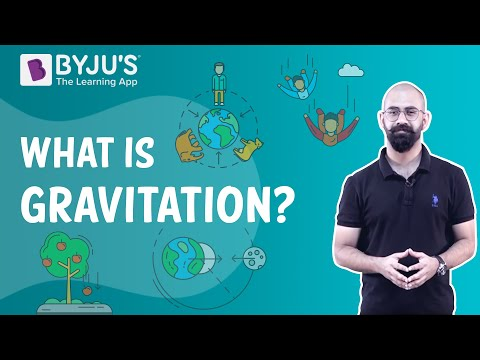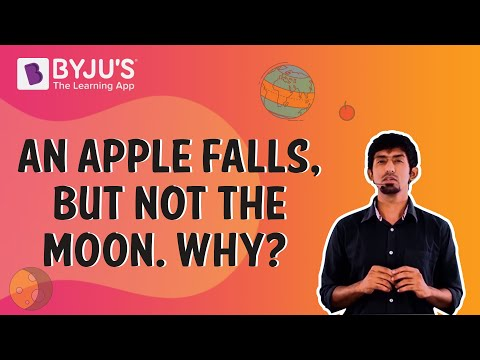# Gravitation Class 9 CBSE Notes - Chapter 10

## The Universal Law of Gravitation

Newton’s Law of gravitation states that every object in the universe attracts every other object by a force that is directly proportional to the product of their masses and inversely proportional to the square of the distance between them.

$$\begin{array}{l}⇒F ∝ M * m\end{array}$$

$$\begin{array}{l}F \alpha \frac{1}{d^{2}}\end{array}$$

$$\begin{array}{l}F = G \frac{Mm}{r^{2}}\end{array}$$
where G is the universal gravitation constant.

⇒ Value of G =

$$\begin{array}{l}6.673*10^{^{-11}}Nm^{2}Kg^{-2}\end{array}$$To know more about Universal Law of Gravitation, visit here.

### Acceleration due to Gravity

$$\begin{array}{l}F = mg and also F = G\frac{Mm}{R^{2}}\end{array}$$

$$\begin{array}{l}g= G\frac{Mm}{R^{2}}\end{array}$$

⇒Plug the values of G =

$$\begin{array}{l}6.673*10^{^{-11}}Nm^{2}Kg^{-2}\end{array}$$

⇒ M(mass of Earth) = 6 * 1024 kg  and R= 6 * 106 m , to get the value of gas ≈

$$\begin{array}{l}9.8ms^{-2}\end{array}$$
.

This is the acceleration due to gravity and the acceleration felt by any freely falling body towards the Earth.
Value of g keeps changing due to the variation of Earth’s radius.To know more about Acceleration due to Gravity, visit here.

### The Moon’s Falling – Moon’s revolution around Earth

The moon revolves around the Earth due to centripetal force, which is the force of gravity of the Earth.  If the force of attraction between the Earth and moon ceases, then the moon will continue to travel in a straight line path tangential to its orbit around the Earth.

#### For More Information On Why Moon Does Not Fall, Watch The Below Video:### Centripetal force

When a body undergoes circular motion, it experiences a force that acts towards the centre of the circle. This centre-seeking force is called a centripetal force.

To know more about Centripetal Force, visit here.

### Free Fall and Motion

When an object is under free fall, acceleration due to gravity is constant at g =

$$\begin{array}{l}9.8ms^{-2}\end{array}$$
.

Value of g does not depend on mass i.e any object big or small experiences the same acceleration due to gravity under free fall. All three equations of motion are valid for freely falling objects as it is under uniform motion.

The sign of convention → towards earth g is +ve / away from earth g is -ve.

To know more about Free Fall, visit here.

### Weight and Mass

Mass of an object is the measure of its inertia and is constant throughout the universe. Weight of an object keeps changing as the value of g changes. Weight is nothing but a force of attraction of the Earth on an object =  mg.
Weight of an object on the Moon is

$$\begin{array}{l}\frac{1}{6}\end{array}$$
times the weight on Earth.

To know more about Weight and Mass, visit here.

### Thrust and Pressure

Force acting on an object perpendicular to the surface is called thrust. Effect of thrust depends on the area of contact. The pressure is thrust per unit area. SI unit is the pascal (Pa). Force acting on a smaller area applies more pressure than the same force acting on a larger area.

#### For More Information On Thrust and Pressure, Watch The Below Video:To know more about Thrust and Pressure, visit here.

### Pressure in fluids

The pressure exerted by a fluid in a container is transmitted undiminished in all directions on the walls of the container.

Archimedes’ Principle – Why objects float or sink

The upward force exerted by a fluid on an object is known as upthrust or buoyant force.

The magnitude of buoyancy depends on the density of the fluid.  If the density of an object is less than the fluid, it will float. If the density of the object is greater than the fluid, it will sink.

According to the Archimedes’ principle, when a body is immersed fully or partially in a fluid, it experiences an upward force that is equal to the weight of the fluid displaced by it.

### Relative Density

Relative density = Density of a substance/ Density of water
To know more about Relative Density, visit here.

### The Story of Gravity – Introduction to Gravitation: Kepler’s laws

The orbit of a planet is an ellipse with the sun as its foci.  The line joining the planets and the sun sweeps equal areas in equal intervals of time. Cube of a mean distance of a planet from the sun ∝ Square of orbital time period T.

$$\begin{array}{l}r^{3}\alpha T^{2}\end{array}$$

To know more about Kepler’s laws, visit here.

## Frequently Asked Questions on CBSE Class 9 Science Notes Chapter 10: Gravitation

### What is gravity?

Gravity is a natural phenomenon by which all masses and energy are attracted to one another and to Earth.

### What is relative density?

The ratio of the density of a substance to the density of a standard substance under specified conditions.

### What is meant by free fall?

When an object falls under the sole influence of gravity, the object is said to be under ‘free fall’.

#### 1 Comment

1. Pooja devi

I’ve been using this app since a long time and it’s of a lot of help to me